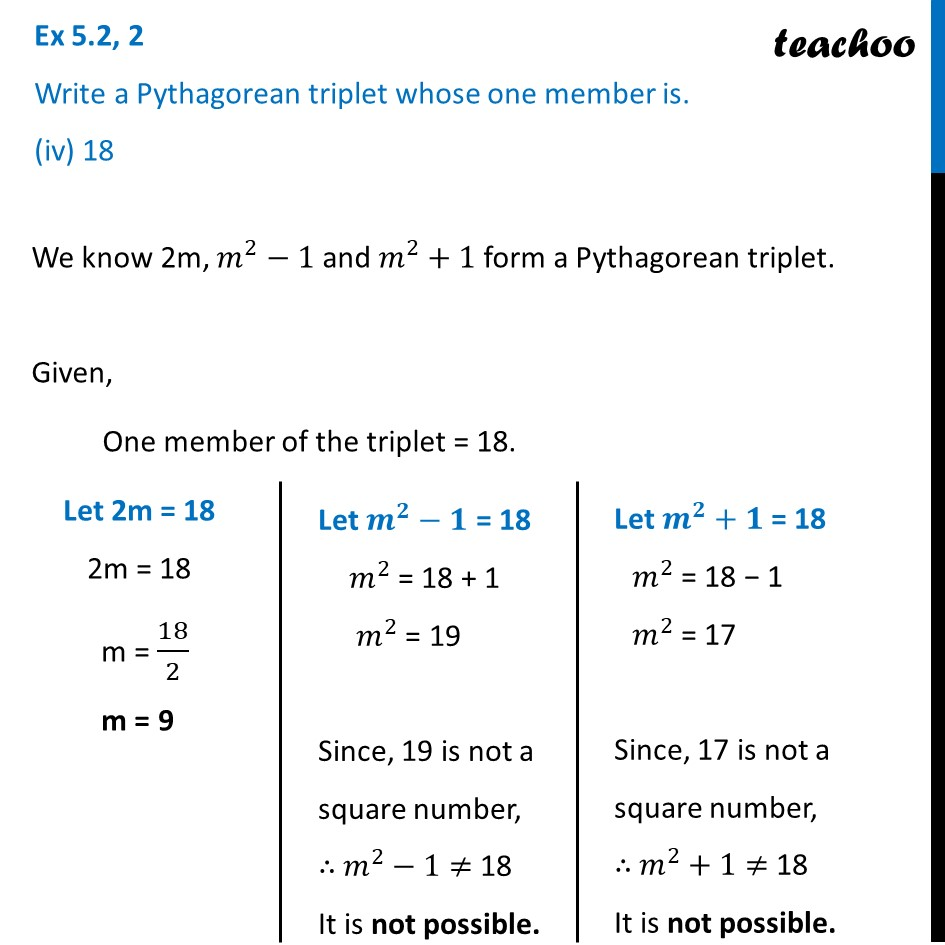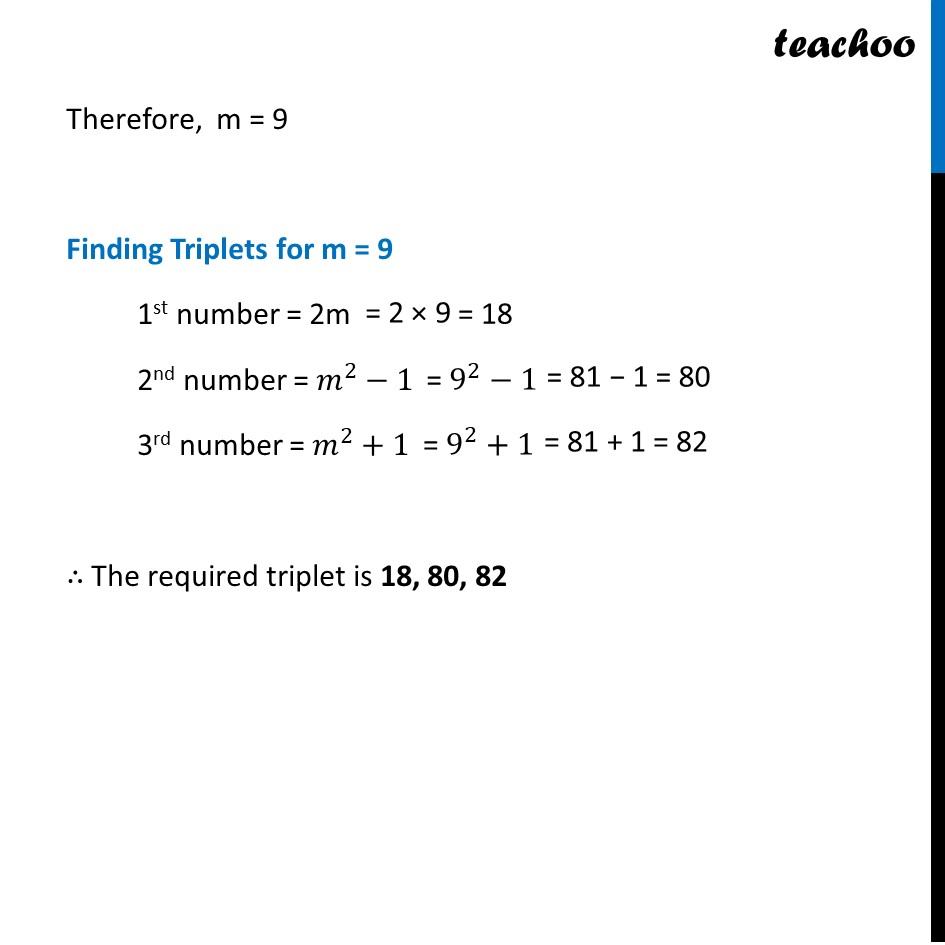Ex 5.2

Chapter 5 Class 8 Squares and Square Roots
Serial order wiseLearn in your speed, with individual attention - Teachoo Maths 1-on-1 Class

### Transcript

Ex 5.2, 2 Write a Pythagorean triplet whose one member is. (iv) 18We know 2m, 𝑚^2−1 and 𝑚^2+1 form a Pythagorean triplet. Given, One member of the triplet = 18. Let 2m = 18 2m = 18 m = 18/2 m = 9 Let 𝒎^𝟐−𝟏" = 18" 𝑚^2 = 18 + 1 𝑚^2 = 19 Since, 19 is not a square number, ∴ 𝑚^2−1 ≠ 18 It is not possible. Let 𝒎^𝟐+𝟏 = 18 𝑚^2 = 18 − 1 𝑚^2 = 17 Since, 17 is not a square number, ∴ 𝑚^2+1 ≠ 18 It is not possible. Therefore, m = 9 Finding Triplets for m = 9 1st number = 2m 2nd number = 𝑚^2−1 3rd number = 𝑚^2+1 ∴ The required triplet is 18, 80, 82